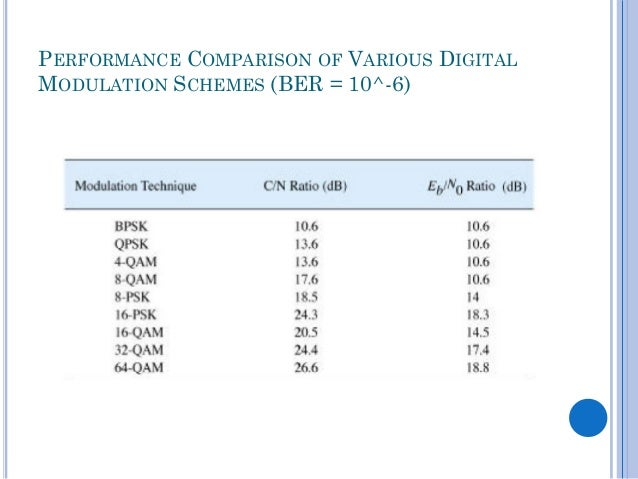# Digital Communication System – Comparison

DIGITAL COMMUNICATION SYSTEM – COMPARISON (ASK- FSK- PSK- QAM):

 S.No Parameter PSK DPSK QPSK QAM FSK ASK 1 Modulation of Phase Phase Phase Amplitude and Phase Frequency Amplitude 2 Equation of transmitted signal s(t)=b(t) √2Ps    (cos  2∏f0t) s(t)=b(t) √2Ps    (cos  2∏f0t) s(t)=b(t) √2Ps (cos  2∏f0t+(2m+1) ∏ / 4       m=0,1,2,3 s(t)= k1√0.2Ps  (cos  2∏f0t) + k2√0.2Ps  (sin  2∏f0t) s(t)= √2Ps    [cos  2 (∏f0+d(t)Ω)t f-frequency shift s(t)= √2Ps    (cos  2∏f0t) for symbol ’1’=0 for symbol ‘0’ 3 Bits per symbol One One Two N One One 4 Number of possible symbols M= 2N Two Two Four M= 2N Two Two 5 Detection method Coherent Non-Coherent Coherent Coherent Non-Coherent Coherent 6 Minimum Euclidean distance 2√Eb 2√Eb √0.4Es for M=16 √2Eb √Eb 7 Minimum Bandwidth(BW) 2fb fb fb 2fb/N 4fb 2fb 8 Symbol duration(Ts) Tb 2Tb 2Tb NTb Tb Tb